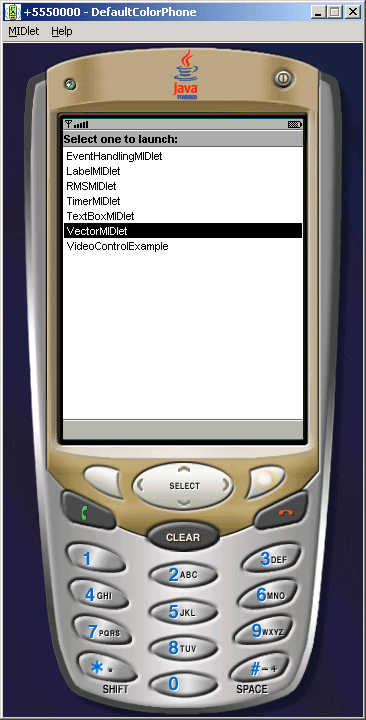Tutorials

# J2ME Vector Example

This application illustrates how we can use Vector class. In this example we are using the vector class in the canvas form.

This application illustrates how we can use Vector class. In this example we are using the vector class in the canvas form.

# J2ME Vector Example

This application illustrates how we can use Vector class. In this example we are using  the vector class in the canvas form. The vector class contains components that can be accessed using an integer index. The following methods are in this class:

• capacity()
• contains(Object elem)
• copyInto(Object[] anArray)
• elementAt(int index)
• elements()
• ensureCapacity(int minCapacity)
• firstElement()
• indexOf(Object elem)
• indexOf(Object elem, int index)
• insertElementAt(Object obj, int index)
• isEmpty()
• lastElement()
• lastIndexOf(Object elem)
• lastIndexOf(Object elem, int index)
• removeAllElements()
• removeElement(Object obj)
• removeElementAt(int index)
• setElementAt(Object obj, int index)
• setSize(int newSize)
• toString()
• trimToSize()

You can use the above method by accessing vector class. The Application is as follows:VectorMIDlet.java

```import java.io.*;
import java.util.*;
import javax.microedition.midlet.*;
import javax.microedition.lcdui.*;

public class VectorMIDlet extends MIDlet{
private VectorCanvas vector;
private Display display;

public VectorMIDlet() {
vector = new VectorCanvas();
display = Display.getDisplay(this);
}

public void startApp() {
display.setCurrent(vector);
}

public void pauseApp() {}

public void destroyApp(boolean unconditional) {}
}

class VectorCanvas extends Canvas{
public void paint(Graphics g){
Vector vector = new Vector(3, 2);

System.out.println("Size of Vector: " + vector.size());
System.out.println("Capacity of Vector: " + vector.capacity());

System.out.println("Capacity after four additions: " + vector.capacity());

System.out.println("Current capacity: " + vector.capacity());

System.out.println("Current capacity: " + vector.capacity());

System.out.println("Current capacity: " + vector.capacity());

System.out.println("First element: " + (Integer)vector.firstElement());
System.out.println("Last element: " + (Integer)vector.lastElement());

if(vector.contains(new Integer(3))){
System.out.println("Vector contains 3.");
}

Enumeration vEnum = vector.elements();
System.out.println("\nElements in vector:");

while(vEnum.hasMoreElements()){
System.out.print(vEnum.nextElement() + " ");
}
System.out.println();
}
}
```